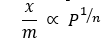The variation in the amount of gas adsorbed by the adsorbent with pressure at constant temperature can be expressed by means of a curve termed as adsorption isotherm.

A mathematical equation which describes the relationship between pressure (p) of the gaseous adsorbate and the extent of adsorption at any fixed temperature is called adsorption isotherms.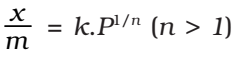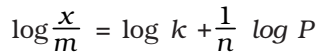where, x is the weight of the gas adsorbed by m gm of the adsorbent at a pressure P, thus x/m represents the amount of gas adsorbed by the adsorbent per gm (unit mass), k and n are constant at a particular temperature and for a particular adsorbent and adsorbate (gas), n is always greater than one, indicating that the amount of the gas adsorbed does not increase as rapidly as the pressure.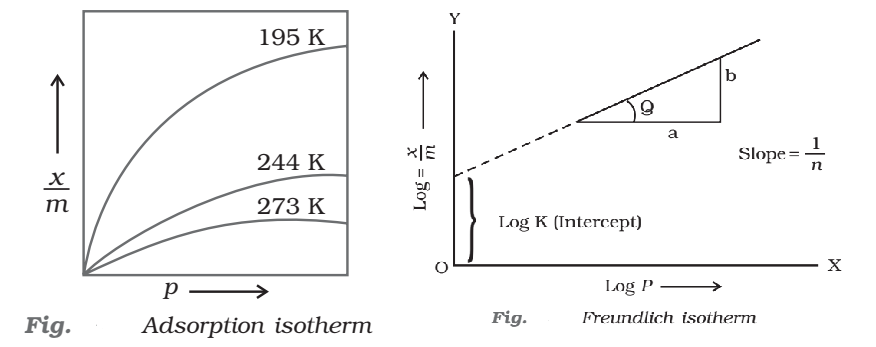At low pressure, the extent of adsorption varies linearly with pressure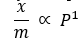At high pressure, it becomes independent of pressure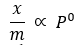At moderate pressure x/m depends upon pressure raised to powers